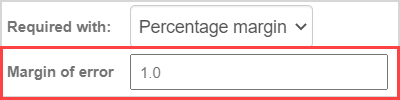# Author a numeric question

A numeric question compares a student's numerical response with a defined numerical answer.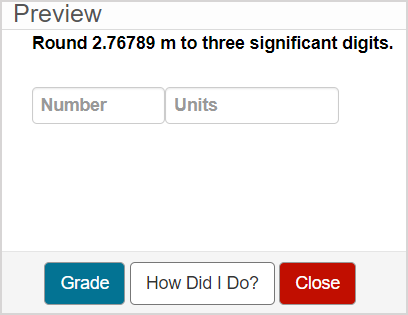Numeric questions can be authored to accept the following numeric formats:

• Pure numbers in decimal form
• Pure numbers in scientific notation
• Dollar values
• Arithmetic and expressions
• Negative signs or parentheses to indicate negative values
• Numbers with a unit of measure

This question type automatically accepts equivalent responses, and the accepted accuracy and tolerance of student numerical responses can be controlled by defining additional grading details for the question.

TIP: You can include an algorithm in your numeric question! Check out Author an algorithmic question.

TIP: All questions are authored using the Question Editor. Check out Author a question to learn how to access the Question Editor.

## To author a numeric question

To author a numeric question from the Question Editor:

1. Enter the question statement in the Question Text pane of the Question Editor.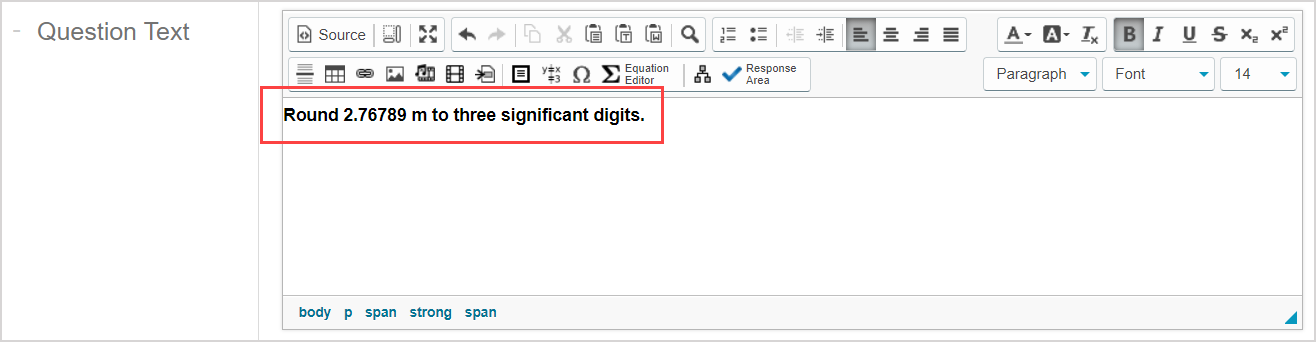TIP: Check out Use the Visual Editor for details on how to use this toolbar to the fullest to control the visual appearance of your numeric question text.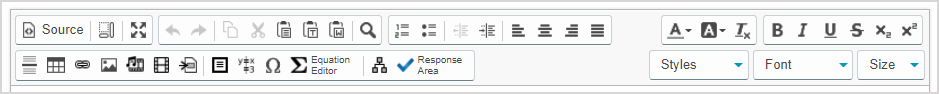1. Click Response Areato select the type of response area for this part of the question.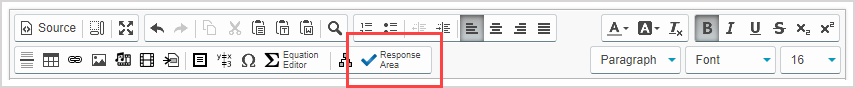NOTE: A question can have multiple question parts. Each question part have a different type of response area. Example — A question could have the first response area as a numeric response area, and a second response area as multiple choice.

1. Click Numeric from the list of response area types.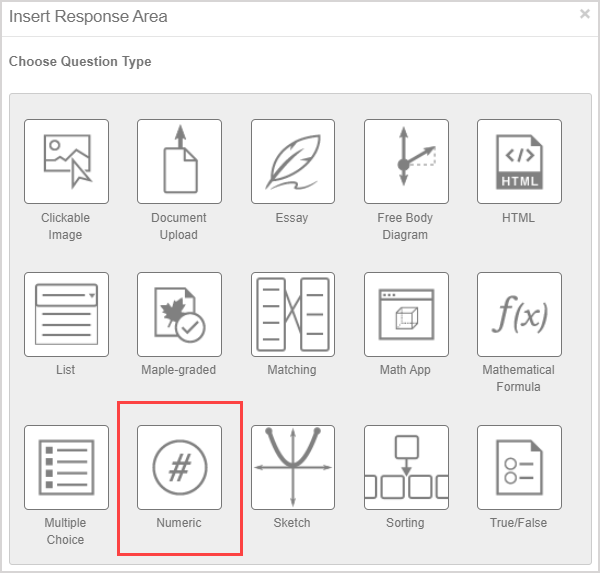1. Assign a weight to the response area. The default response area weighting is 1.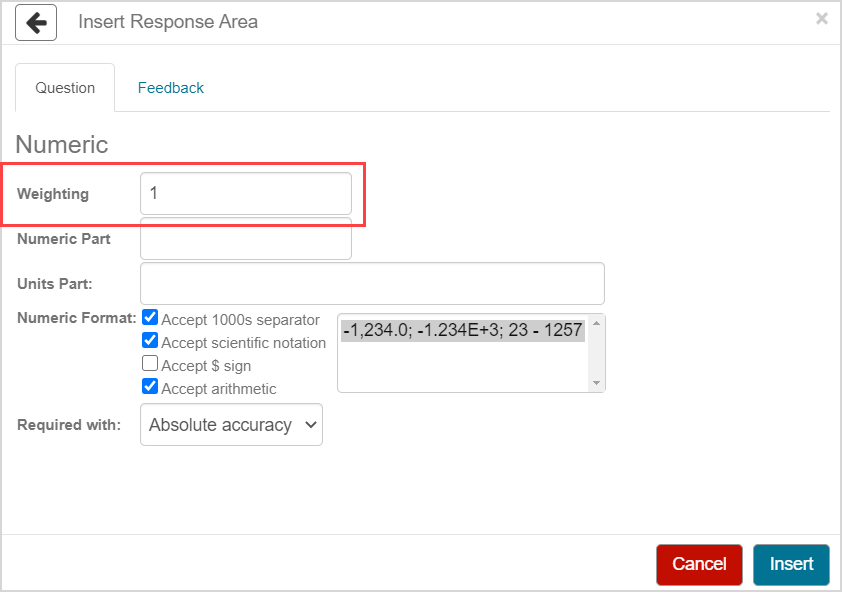TIP: The response area weight can be any integer greater than 0. Response area weighting is proportionate to the question total. Example — A question with two response areas could have the first response area weighted as 2, and the second response area weighted as 4. This means that the second response area will be worth twice as much as the first response area when calculating the total grade for the question.

1. Enter the numeric component of the correct answer in the Numeric Part field.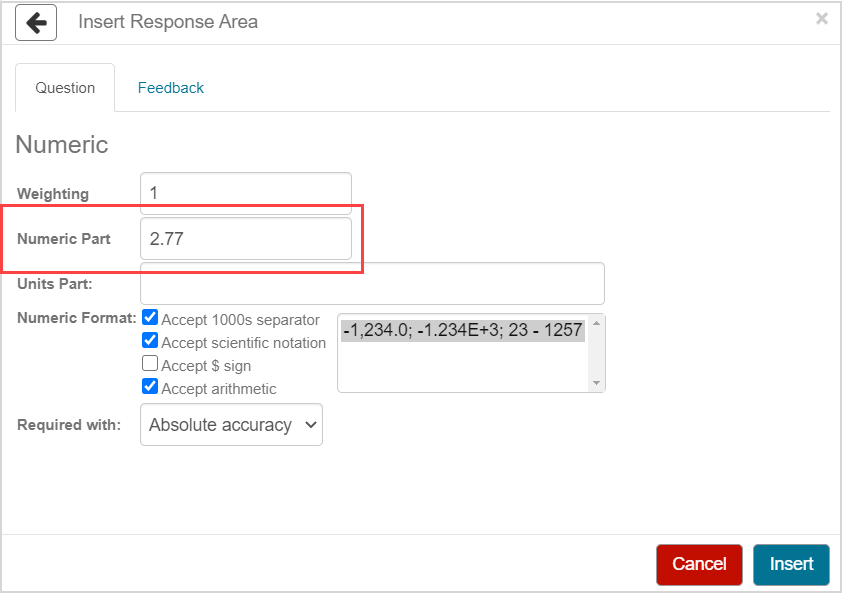NOTE: Equations and algebraic formulas can't be used as the correct answer.

1. (Optional) Enter the unit of measure for your correct answer in the Units Part field.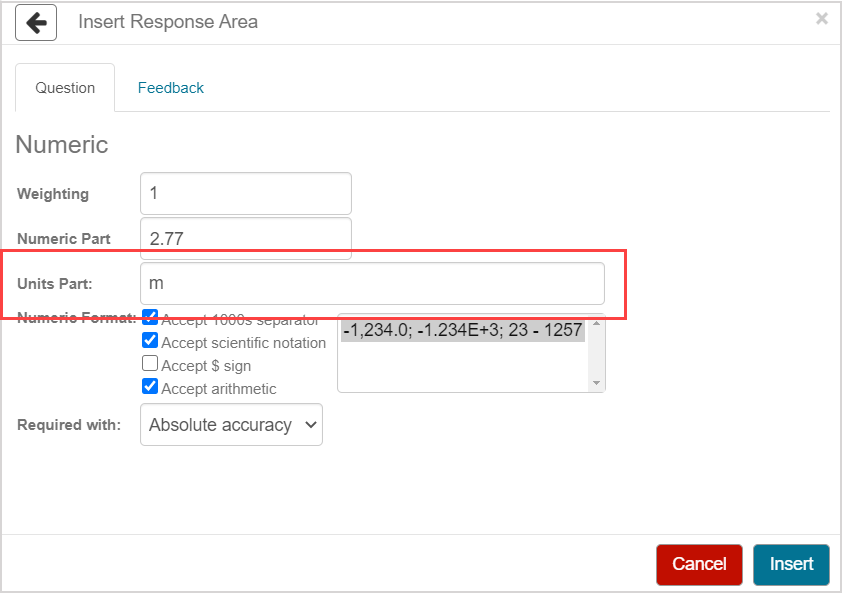NOTE: Only specific units of measure are recognized by Möbius. Check out Author a question with physical units for a comprehensive list of physical units that are recognized by Möbius.

NOTE: The Units field will be visible to students indicating that a unit of measure is required in their response if you've entered a value in the Units Part field during question authoring.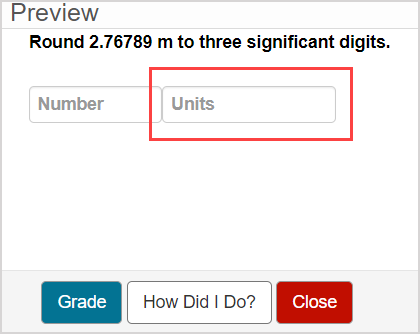Students are provided with a Units Help link within the question if you've entered a value in the Units Part field during question authoring. Students can click within the Units answer field to reveal the Units Help link which contains details about the allowed units of measure and their equivalents.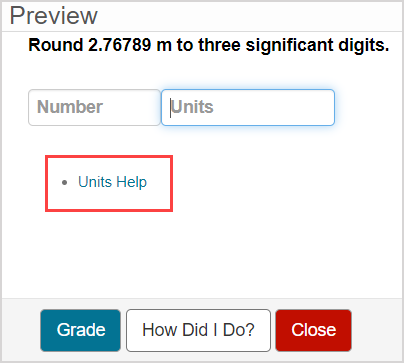The Units field won't be visible and answers are dimensionless if no value is entered in the Units Part field during question authoring.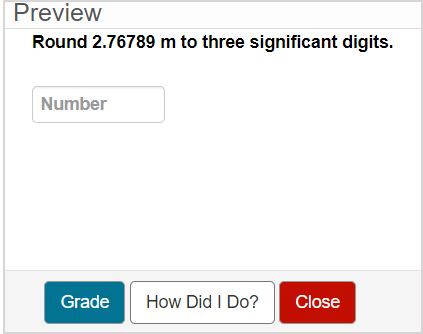1. Select the Numeric Format check boxes that you want to apply to your question. Check out the Numeric format properties section of this help topic for details on these options.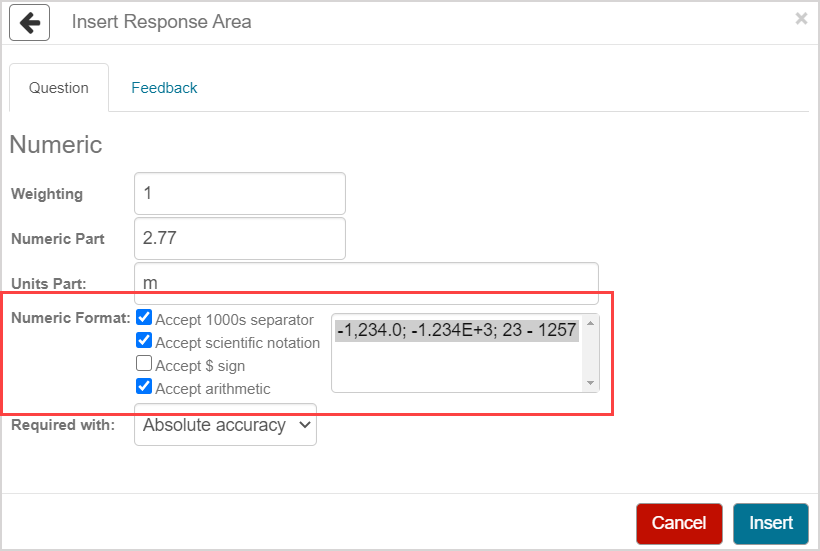1. Make a selection from the Required with drop-down menu to determine the accepted accuracy and allowed tolerance of student responses for your question. Check out the Grading details for numeric questions section of this help topic for details on these options.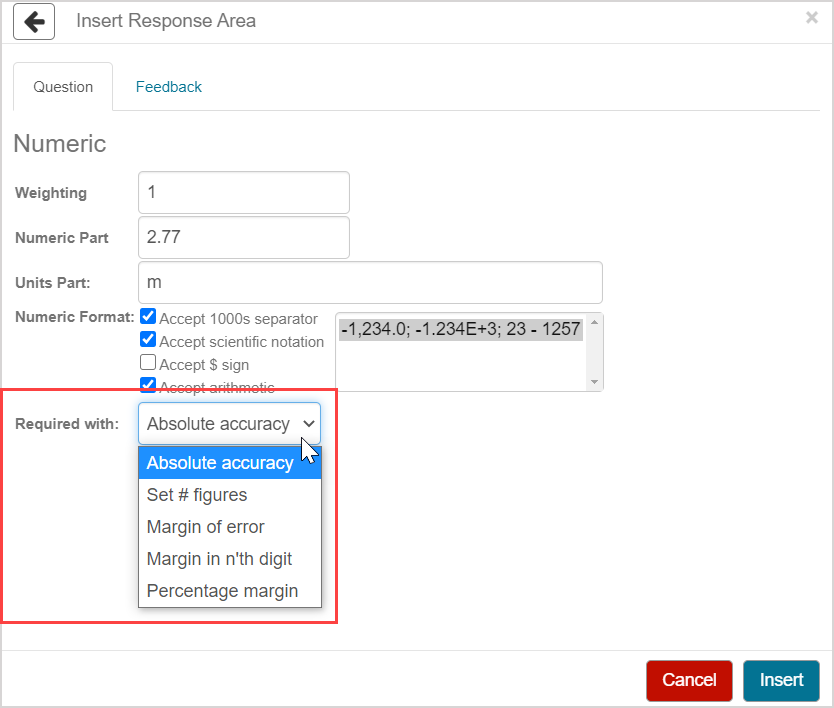1. Click Insert to add this response area to the question.1. The response area is inserted into your question.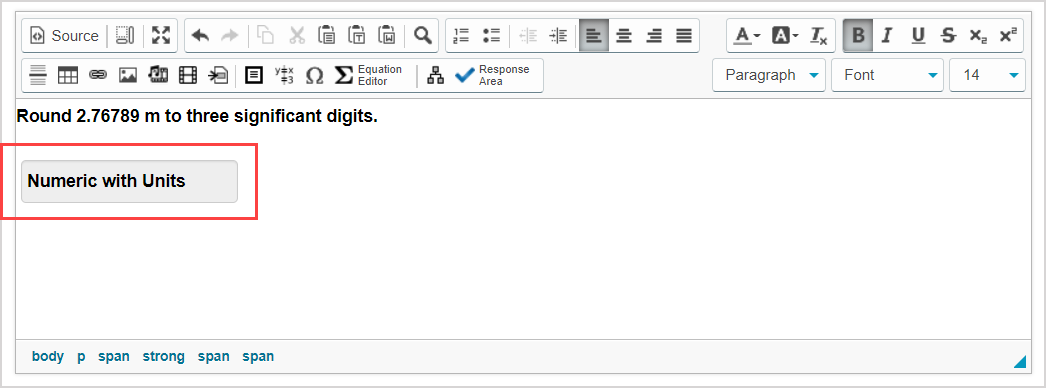1. Click Preview to trial your new question to ensure that it behaves as expected.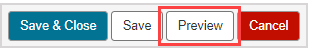TIP: You first have to click the save iconif you're creating this question from within an Activity Editor. After the question has been saved, you can then click the preview icon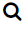to ensure that your new question behaves as expected.

1. Click Save to save your new question. Your new question is now stored in the Questions pane of the Content Repository.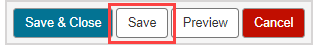TIP: Click the save iconif you're creating this question from within an Activity Editor. This saves your question to the activity and the Content Repository.

## Numeric format properties

The following numeric format options are available when deciding what type of responses will be accepted from your students:

NOTE: You can select any assortment of Numeric Format check boxes for your question.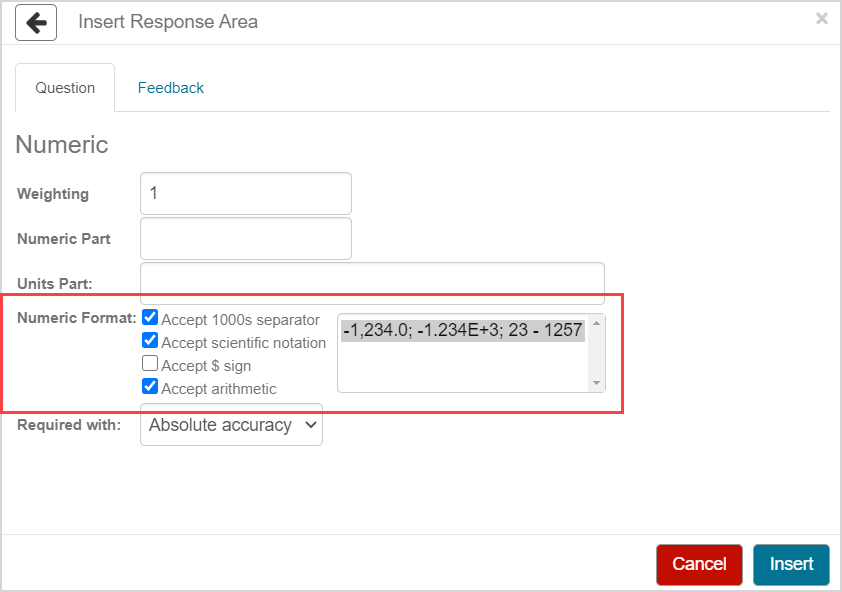Numeric format Example of accepted response Description
Accept 1000s separator 1,234

A comma as the thousands separator is accepted in student responses.

Selected by default.

Accept scientific notation 1.234E+3

Scientific notation is accepted in student responses.

Selected by default.

Accept \$ sign \$1234

A leading dollar sign (\$) is accepted in student responses.

Accept arithmetic 1230+4

Arithmetic operators (Example+, -, *, /, (), and ^) are accepted in student responses.

Selected by default.

TIP: If your correct answer includes arithmetic, ensure that the Accept arithmetic numeric format is enabled. Example — If your answer is 1/3 and Accept arithmetic is disabled, students won't be able to submit a correct answer of 1/3.

NOTE: Parentheses can't be accepted to indicate negative values if the Accept arithmetic numeric format is enabled.

TIP: You can make additional selections for each numeric format on how to manage negative values in student responses: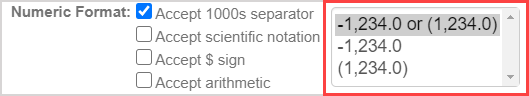Example — When working with the Accept 1000s separator numeric format, you can:

• Accept values with the negative sign (Example-1,234.0) and values enclosed in parentheses as negatives (Example(1,234.0)).
• Only accept values with the negative sign
• Only accept bracketed values

NOTE: Students are provided with a Number Help link within the question based on the selections you make from the Numeric Format field during question authoring. Students can click within the Number answer field to reveal the Number Help link which contains details about the allowed numeric formats.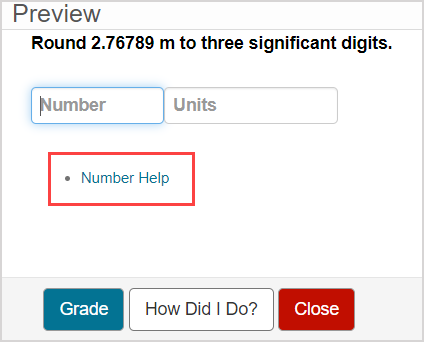## Grading details for numeric questions

The options of the Required with drop-down menu allow you to define the accepted accuracy and allowed tolerance of student responses for your question: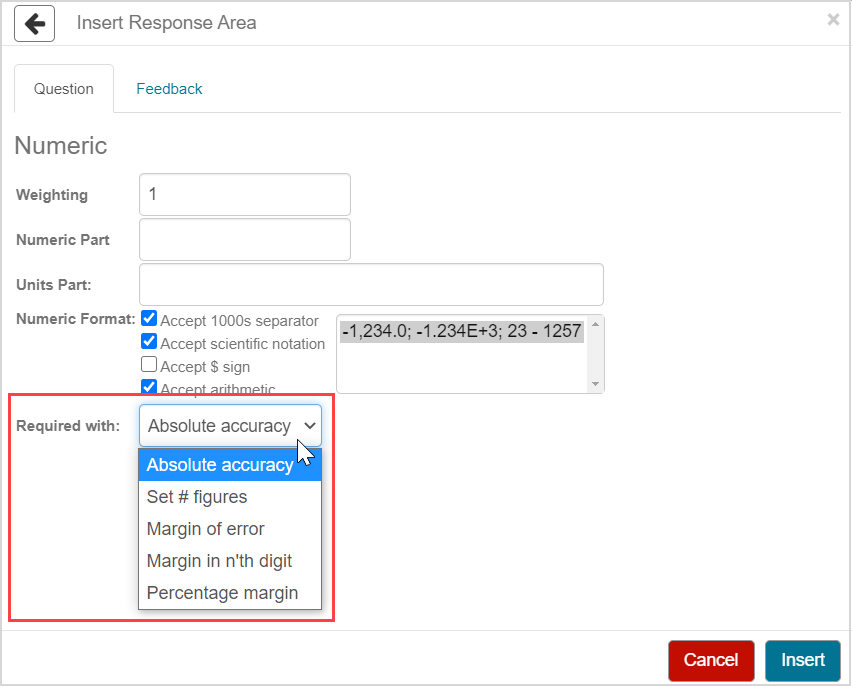NOTE: Regardless of the accepted numerical accuracy and tolerance, a student's response will be graded as incorrect if their units component is incorrect or missing (if the question requires a units component).

Accuracy or tolerance option Example Description
Absolute accuracy
• Correct student response: 1234
• Correct student response: \$1,234
• Correct student response: 1233+1
• Correct student response: 123.4E+1
• Incorrect student response: 1234.1

The student's response must be an exact match (or be equivalent) up to floating-point limits (approximately eight decimal places).

Set # figures
• Correct answer requiring four significant figures: 123.4
• Correct student response: 123.4
• Correct student response: 1234E-1
• Partially correct student response: 123.40
• Incorrect student response: 123

Enter how many significant figures must be in the student's response using the # figures field.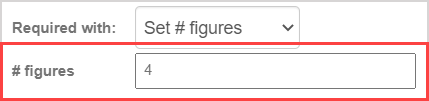IMPORTANT: Your defined correct answer in the Numeric Part field should have the same number of significant figures as defined by the Set # figures grading property. Example — If the correct answer is 123.4, then 4 should be the value entered in the # figures field.

NOTE: A partial grade of 50% is awarded if the Set # figures grading property is defined and a student's response is mathematically correct but contains the incorrect number of significant figures.

NOTE: The Set # figures grading property can't be paired with the Accept arithmetic numeric format.

TIP: When working with the Set # figures grading property and algorithms, it's recommended that you use the sig algorithmic function to create algorithmic variables. By using these variables in the question statement, feedback, hints, and answer, you ensure that values are displayed with the correct number of significant digits. Check out View available functions for algorithmic questions.

Margin of error
• Within 0.1 of correct answer: 123.4
• Correct student response: 123.4
• Correct student response: 123.5
• Correct student response: 123.3
• Incorrect student response: 123.6
• Incorrect student response: 123.2

The student's response must be within +/- (inclusive) of the value defined in the Margin of Error field.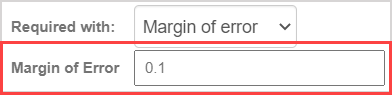Margin in n'th digit
• Within 1 of correct answer at the fifth digit: 123.45
• Correct student response: 123.45
• Correct student response: 123.46
• Correct student response: 123.44
• Partially correct student response: 123.455
• Incorrect student response: 123.445
• Incorrect student response: 123.47
• Incorrect student response: 123.5

The student's response must be within +/- (inclusive) of the value defined in the Margin of Error field at the significant digit specified in the Digit field.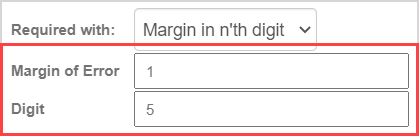NOTE: A partial grade of 50% is awarded if the Margin in n'th digit grading property is defined and a student's response is mathematically correct but contains the incorrect number of significant figures.

NOTE: The Margin in n'th digit grading property can't be paired with the Accept arithmetic numeric format.

Percentage margin
• Within 1.0% (1.234) of correct answer: 123.4
• Correct student response: 124.6
• Correct student response: 122.166
• Incorrect student response: 124.7
• Incorrect student response: 124.635
• Incorrect student response: 122.09

The student's response must be within +/- (inclusive) percent of the defined value in the Margin of error field.